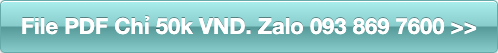rating

# Successful Traders Size their Positions why and how

Successful Traders Size their Positions why and how.

Đặt in thành sách tại HoaXanh hay mua file PDF:• 50,000đ
• Mã sản phẩm: SU2206
• Tình trạng: 1

Chapter 1 - Three Requirements for Trading Success

Managing the position and the portfolio

Summary of the three parts of trading success

Chapter 2 - Strategy Versus System

Chapter 3 - Why Control Position Size?

Chapter 4 - Controlling Risk of Your Initial Position

Introduction to stock simulations on risk allocation

Various simulations using risk allocation percentages - stocks

Analysis of various simulations on risk – stocks

Introduction to futures simulations on risk

Various simulations using risk allocation percentages – futures

Analysis of various simulations on risk - futures

Chapter 5 - Controlling Volatility of Your Initial Position

A simple way to calculate volatility in a position

Sizing your initial position using volatility

Introduction to stock simulations using volatility allocation

Various simulations using volatility allocation percentages - stocks

Analysis of various simulations on volatility – stocks

Introduction to futures simulations using volatility sizing

Simulations using volatility allocation percentages – futures

Analysis of various simulations on volatility - futures

Chapter 6 - Controlling Capital/Margin Your Initial Position

Chapter 7 – Combining the Approaches Makes a Lot of Sense

Analysis of various risk/volatility combinations on – stocks

Introduction to futures simulations using risk/volatility sizing

Various simulations using risk/volatility – futures

Analysis of various simulations on volatility - futures

Chapter 8 – Various Forms of Equity in Your Portfolio

Starting with a small account equity

Chapter 9 – Now That You Are in the Position, What Now?

Ongoing position risk allocation limit

Introduction to futures simulations using ongoing risk sizing

Various simulations using ongoing risk limits – futures

Analysis of various simulations on ongoing risk limits - futures

Ongoing position volatility allocation

Introduction to futures simulations using ongoing volatility sizing

Various simulations using ongoing risk limits – futures

Analysis of simulations on ongoing volatility limits - futures

Ongoing position margin allocation

Chapter 10 - Combining Ongoing Risk and Volatility Limits – Futures

Introduction to simulations using ongoing risk/volatility sizing

Simulations using ongoing risk/volatility limits – futures

Analysis of simulations of Ongoing risk/volatility limits - futures

Chapter 11 - Managing Total Portfolio Risk

Simulations using ongoing portfolio risk limits – futures

Chapter 12 – How Far We’ve Come

Chapter 13 - “Scaling Out” Versus “Right-Sizing”

Chapter 14 – Where to Dial in Your Exposures

Shooting for the moon is not an option

Here's a place where less is more

Chapter 15 – Mr. Serenity’s Thoughts on the Sizing “Sweet Spot”

Không có đánh giá nào cho sản phẩm này.

## Viết đánh giá

Vui lòng đăng nhập hoặc đăng ký trước khi đánh giá